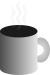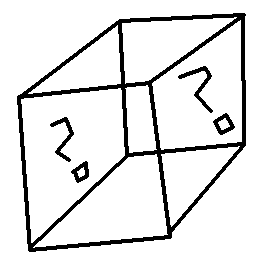#Coffee SpaceListen:

## Protocol ImprovementsPreview Image

This is a write up of some ideas from another project (that I'm not entirely sure I can describe) - and I have since come up with some improvements independently in my own time. They could be useful elsewhere, so I believe they are worth documenting. I am soon to work on a new motor design for RoboCup, so perhaps it would be extremely useful for this use case.

### Problem

You want to transmit some important time critical data that can't be corrupted, if you detect corruption the data must be thrown out. This could be in the form of a single bit being transmitted incorrectly, meaning the entire thing has to be discarded. You are sending blocks of approximately 256 bytes and really don't have much bandwidth to play with.

Pretty challenging huh?

### Error Correction

The method I want to implement is a forward correction matrix, where we store the bit parity. In the example below, an even number of bits is zero, an odd number of bits is one. We sum the columns and rows and store these values in the border.

```0001     1 0 0 0
0002  0  0 1 0 1 <- first row
0003  1  1 1 0 1
0004  0  1 0 0 1
0005  0  1 0 0 1```

For example, we sum the first row, then take modulo two, e.g. `(0 + 1 + 0 + 1) % 2 = 0`. Then if a single bit flips in this matrix, we will get a change in both the row and column, like so:

```0006     1 0(0)0
0007  0  0 1 0 1
0008  1  1 1 0 1
0009 (0) 1 01
0010  0  1 0 0 1```

Here, the square brackets (`[`, `]`) indicate the flipped bit in the matrix and the smooth brackets (`(`, `)`) indicate the check bits that do not correctly calculate the row or column they represent.

To correct, we then simply flip the bit highlighted by the column and row, then correct the sum. As long as you're only expecting a single bit flip, then this works. With two bit flips, it gets a little more complicated:

```0011    (1)0(0)0
0012 (0)1<0>1
0013  1  1 1 0 1
0014 (0)<1>01
0015  0  1 0 0 1```

Now we introduce triangle brackets (`<`, `>`) to represent bits that are not flipped, but are incorrectly flagged by the columns and rows they are on. Because of this, we could end up incorrectly adjusting the matrix as follows:

```0016    (1)0(0)0
0017 (0)1<1>1
0018  1  1 1 0 1
0019 (0)<0>01
0020  0  1 0 0 1```

For that reason, this method is only appropriate for detecting a single bit error in a given matrix - or when errors are repeated in a row or column. When you have more than one error in both the rows and columnsm, there is the possibility you incorrectly adjust the matrix.

Lukcily for us, the nature of transmitting this data tends to mean that if the previous bit had an error, the probability of the next bit having an error is quite high.

The cost of this method for a matrix of size `n^2` is `2n`. For 256 bytes, we have 2048 bits - which doesn't break down so well. We could therefore build a 64x32 matrix (increasing the number of colums as errors are more likely to happen in a row), meaning that have 8 byte (64 bits) correction for columns and 4 byte (32 bits) correction for rows. That is 96 bit correction bits instead of the theoretical best of 92 - so not so bad.

### Going Further

So this is already pretty cool, but what if you want to detect greater number of bit flips in your matrix? Simply doubling the number of check bits quadruples the number of errors you can check for. You have to consider the cost/benefit of doing so of course, given that for 256 bytes of data you are now doubling the amount of checking bytes from 24 to 48.

Another option could be to add another dimension to your data, so that you now correct errors for a given cube. A cube of size 16 x 16 x 8 bits (5 bytes) can account for 256 bytes. By increasing the number of dimensions we dramatically decreased the number of error checking bits required.

The caveat with increasing dimensions is that we see that we end up with this same 'rotation' problem in a given two axis pair if more than one of the axis has more than one error. In some sense we have become less robust to errors, but still have the ability to detect any one given bit flip.

Finally we increase to four dimensions, for a matrix of dimensions 8 x 8 x 8 x 4 bits, which is 3.5 bytes. We then double this to two bits per correction, for a total of 7 bytes. This means that we can now detect up to four bit flips in the matrix of 256 bytes with just 7 bytes of correction data.

This of course should be heavily tested, and debugging a 4D data structure is not so nice, but if the math works out, this is quite a nice error correction methodology for very little cost.

### Future

I'm not entirely sure what to call this extended method, but I think there is some value to it. I will have to put it through some extensive testing, but I think for sure it's quite interesting!

If it holds up, I may look at using this for an up-and-coming motor protocol. Watch this space!